# Flutter Tween Tutorial with Examples

#### View more Tutorials: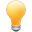Follow us on our fanpages to receive notifications every time there are new articles.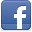Facebook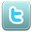Twitter

## 1- Tween

In FlutterTween class tries to simulate a "linear interpolation". So before we talk about it, we need to clarify the concept of interpolation and linear interpolation.
Interpolation is the process of estimating unknown data points that fall between known data points.
To simplify, look at the illustration below. The pink curve includes actual data points, but we only know a few actual data points (in red). The question is, how can we estimate other points?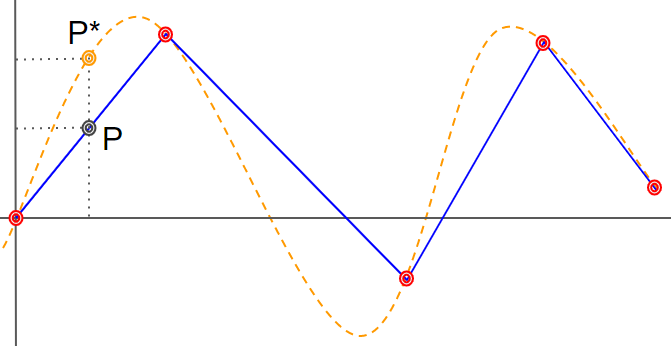Newton's linear interpolation method says that connect points with increasing X-coordinates to create a polylines (Blue) and you can estimate other data points.
According to the illustration above, P is a point on the meandering line (blue), estimated by the linear interpolation method, and P* is the actual data point. Obviously, there is a bit of an error.
Tweet<T>
Back to Tweet<T> class, it simulates a linear interpolation with 2 known data points (starting and ending points). In this case, the polylines is only a straight line.
``````
const Offset(
double dx,
double dy
)
``````
As usual we start with a simple example: A Tweet<T> with the parameter <T> is Offset type. In 0 to 1 time period, an object moves steadily in a straight line from position P0 (2.10) to position P1 (20.4). We can calculate the position of this object at any given time.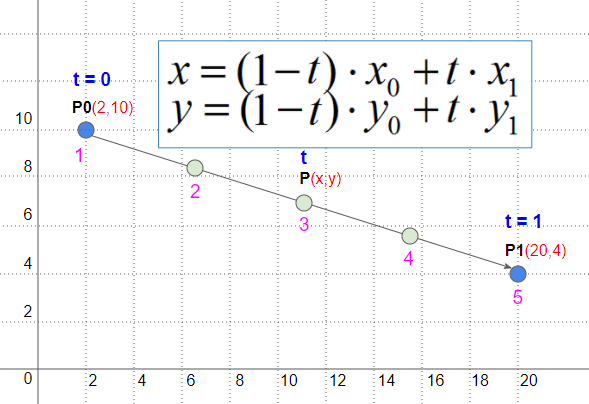``````
Tween<Offset> tween = Tween<Offset>(begin: Offset(2, 10), end: Offset(20,4));

var times = [0.0, 0.25, 0.5, 0.75, 1.0];

for(var t in times) {
Offset point = tween.transform(t);
print("t = " + t.toString() +". x/y = " + point.dx.toString() +"/" + point.dy.toString());
}
``````
Output:
``````
I/flutter (22119): t = 0.0. x/y = 2.0/10.0
I/flutter (22119): t = 0.25. x/y = 6.5/8.5
I/flutter (22119): t = 0.5. x/y = 11.0/7.0
I/flutter (22119): t = 0.75. x/y = 15.5/5.5
I/flutter (22119): t = 1.0. x/y = 20.0/4.0
``````
Thus, just providing 2 data points, Tweet<T> will estimate a lot of other data points, they can be used as different states in an animation process.

## 2- Tween classes

Hierarchy of classes: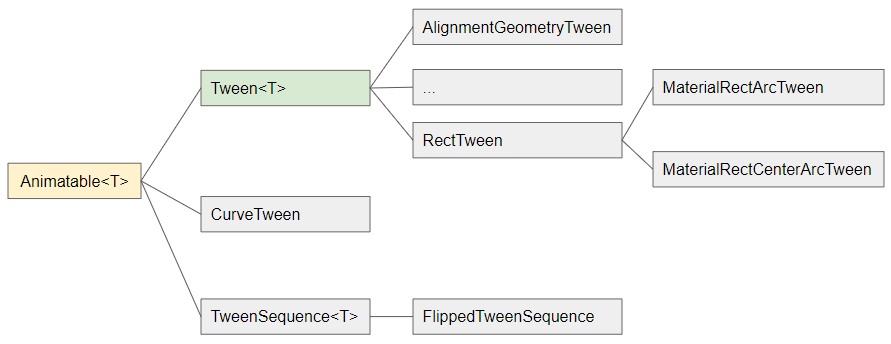List of descendants of Tween class:
1. AlignmentGeometryTween
2. AlignmentTween
4. BorderTween
5. BoxConstraintsTween
6. ColorTween
7. ConstantTween
8. DecorationTween
9. EdgeInsetsGeometryTween
10. EdgeInsetsTween
11. FractionalOffsetTween
12. IntTween
13. MaterialPointArcTween
14. Matrix4Tween
15. RectTween
1. MaterialRectArcTween
2. MaterialRectCenterArcTween
16. RelativeRectTween
17. ReverseTween
18. ShapeBorderTween
19. SizeTween
20. StepTween
21. TextStyleTween
22. ThemeDataTween
Tweet<T> class has quite a few subclasses, some of which are created for specific types of <T> parameter. Example: AlignmentGeometryTween class extends from Tween<AlignmentGeometry>, which is a linear interpolation between 2 AlignmentGeomery(s).

## 3- Tween constructor

``````
Tween<T extends dynamic>(
{T? begin,
T? end}
)
``````
TODO

TODO

## 5- lerp(t)

TODO

#### View more Tutorials:

Maybe you are interested

These are online courses outside the o7planning website that we introduced, which may include free or discounted courses.